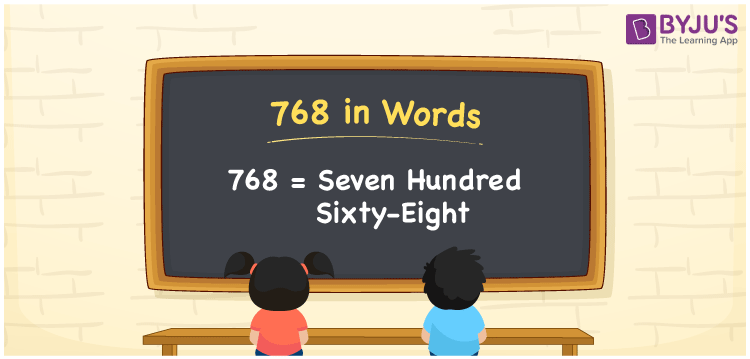# 768 in Words

768 in words is written as Seven Hundred Sixty-eight. The name of number 768 in English is “Seven Hundred Sixty-eight”. The word Seven Hundred Sixty-eight is used in many real-life situations. For example, there are Seven Hundred Sixty-eight students in a classroom. Thus, it is a counting number. Also, to represent the currency equal to 768, we can write it in words as Rupees Seven Hundred Sixty-eight or Seven Hundred Sixty-eight rupees. Therefore, it is necessary to learn the numbers in words, for the ease of understanding and expressing them.

 768 in words Seven Hundred Sixty-eight Seven Hundred Sixty-eight in Numbers 768

## 768 in English Words## How to Write 768 in Words?

If we know the place value of digits of 768, then we can easily express it in words. The place value is basically the position of a digit in a number. 768 is a three-digit number, therefore, we can specify the position of each digit of 768 in a place value chart. In the Indian numbering system, the order of place value of digits from right to left is given by:

 Hundreds Tens Ones 7 6 8

We can write it in expanded form as:

7 × Hundred + 6 × Ten + 8 × One

= 7 × 100 + 6 × 10 + 8 × 1

= 700 + 60 + 8

= 768

= Seven Hundred Sixty-eight

Therefore, 768 in words is written as Seven Hundred Sixty-eight.

768 is a natural number that precedes 769 and succeeds 767.

768 in words – Seven Hundred Sixty-eight

Is 768 an odd number? – No

Is 768 an even number? – Yes

Is 768 a perfect square number? – No

Is 768 a perfect cube number? – No

Is 768 a prime number? – No

Is 768 a composite number? – Yes

## Frequently Asked Questions on 768 in Words

Q1

### How to write 768 in words?

768 in English is written as “Seven Hundred Sixty-eight”.
Q2

### Is 768 an odd number or even number?

768 is an even number because it is wholly divisible by 2.
Q3

### Write Seven Hundred Sixty-eight in numbers.

Seven Hundred Sixty-eight in numbers is 768.